# Major Scale Construction The Major Scale Formulae Constructing

• Slides: 33Major Scale Construction The Major Scale Formulae Constructing The C Major Scale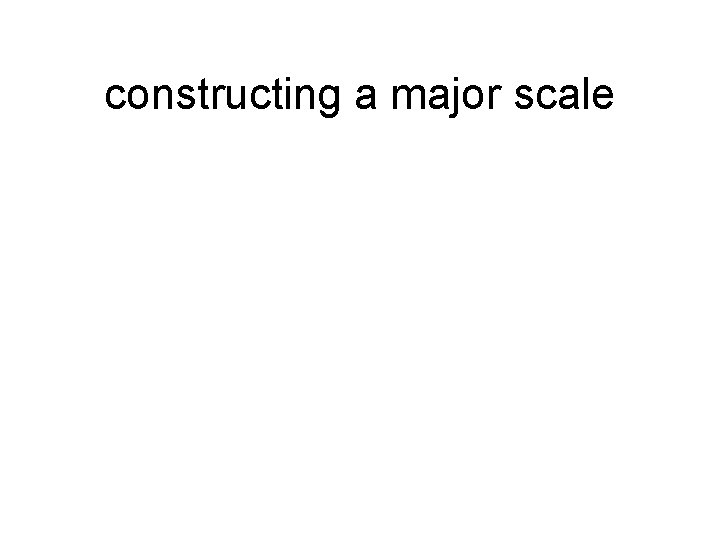constructing a major scale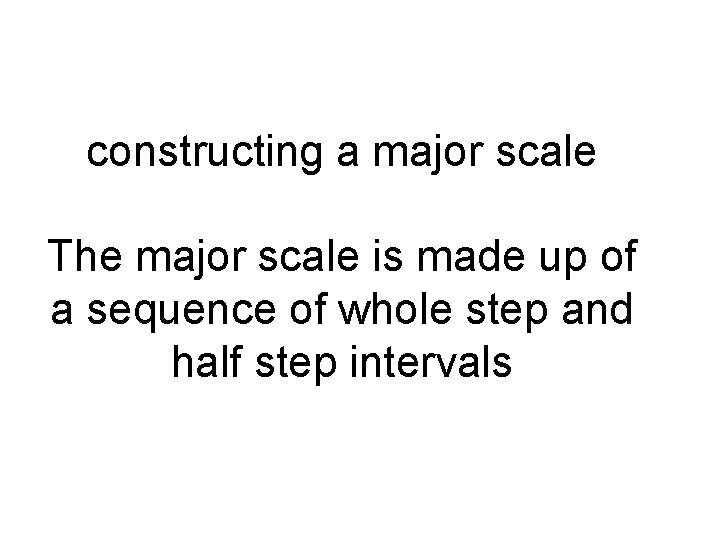constructing a major scale The major scale is made up of a sequence of whole step and half step intervals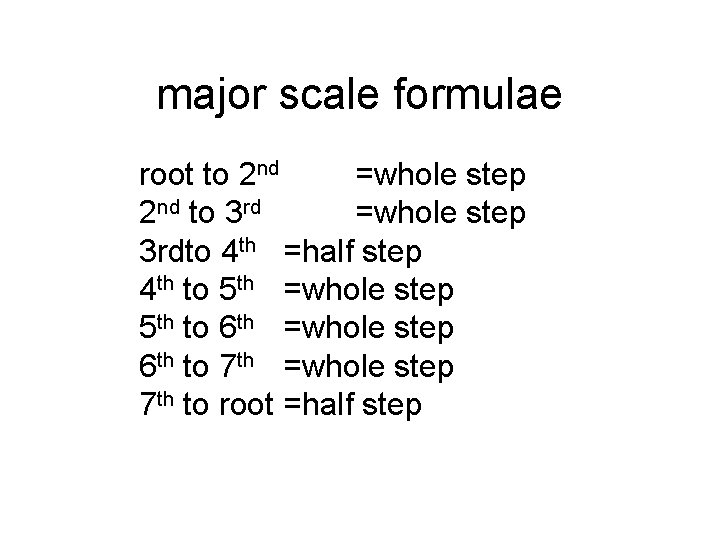major scale formulae root to 2 nd =whole step 2 nd to 3 rd =whole step 3 rdto 4 th =half step 4 th to 5 th =whole step 5 th to 6 th =whole step 6 th to 7 th =whole step 7 th to root =half stepmajor scale formulae whole step half step whole step half step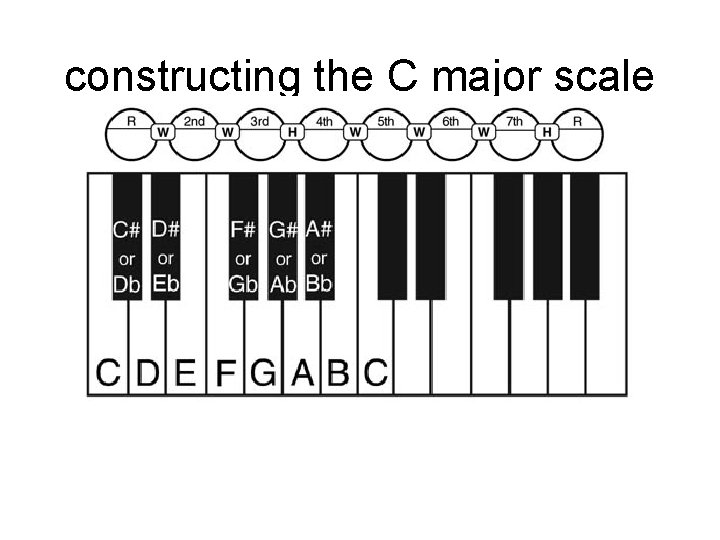constructing the C major scaleThe root note of C Crise a whole step to the note C nd 2the 2 nd = D C Drise a whole step to the 3 rd note C D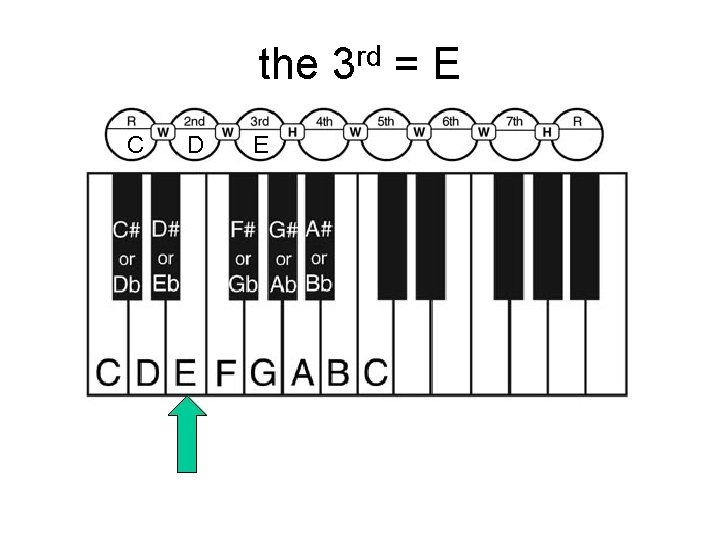the 3 rd = E C D E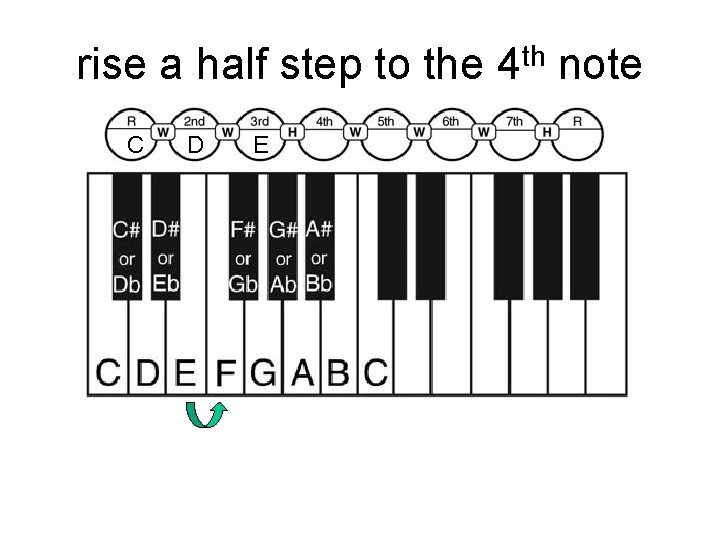rise a half step to the 4 th note C D E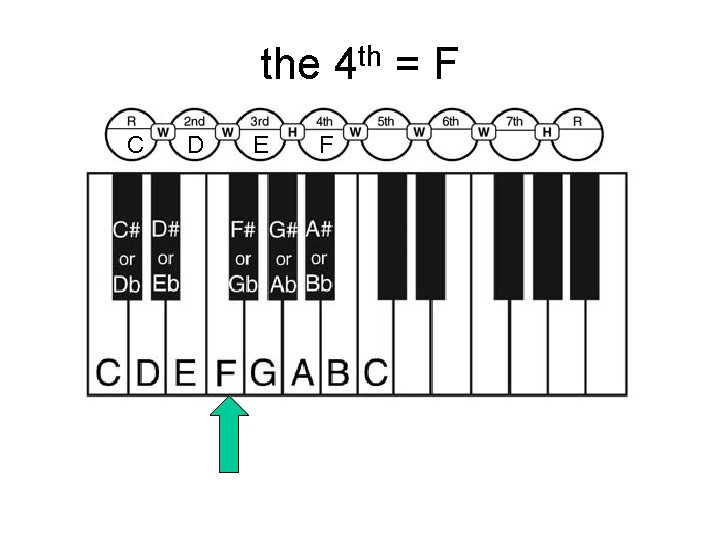the 4 th = F C D E Frise a whole step to the note C D E F G th 5the 5 th = G C D E F G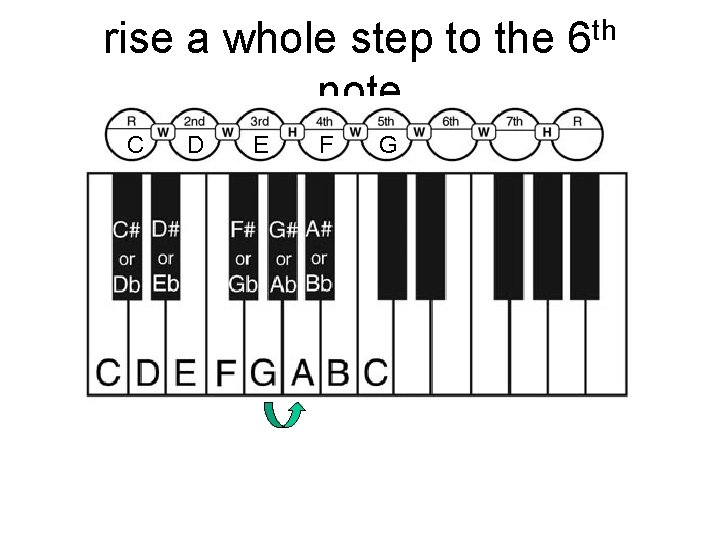rise a whole step to the note C D E F G th 6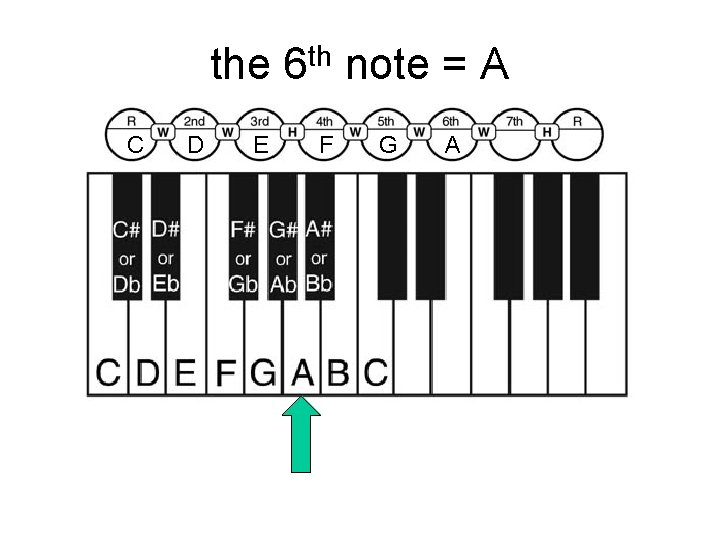the 6 th note = A C D E F G A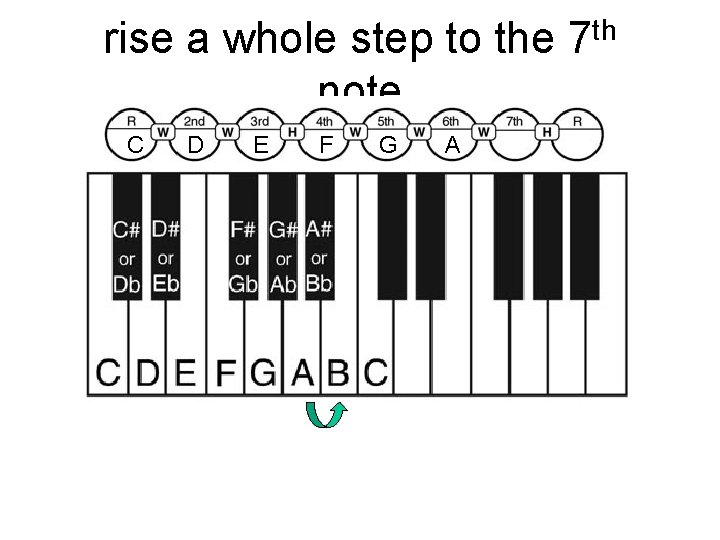rise a whole step to the note C D E F G A th 7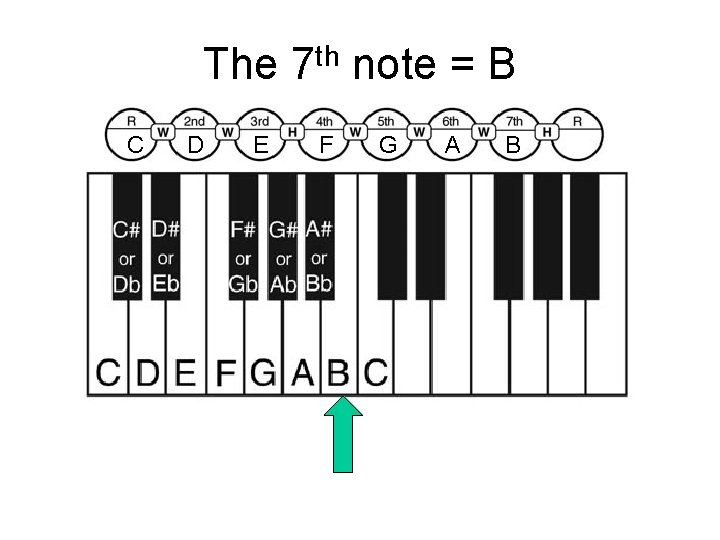The 7 th note = B C D E F G A Brise a half step to the root C D E F G A Bwe are back to C again C D E F G A B C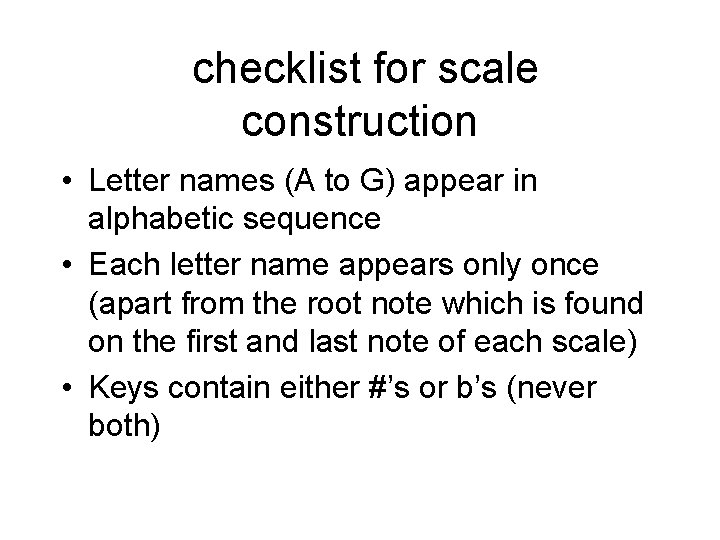checklist for scale construction • Letter names (A to G) appear in alphabetic sequence • Each letter name appears only once (apart from the root note which is found on the first and last note of each scale) • Keys contain either #’s or b’s (never both)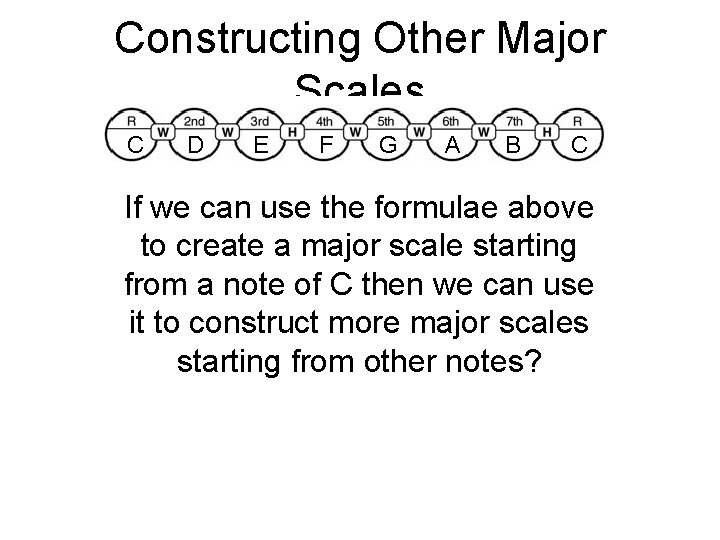Constructing Other Major Scales C D E F G A B C If we can use the formulae above to create a major scale starting from a note of C then we can use it to construct more major scales starting from other notes?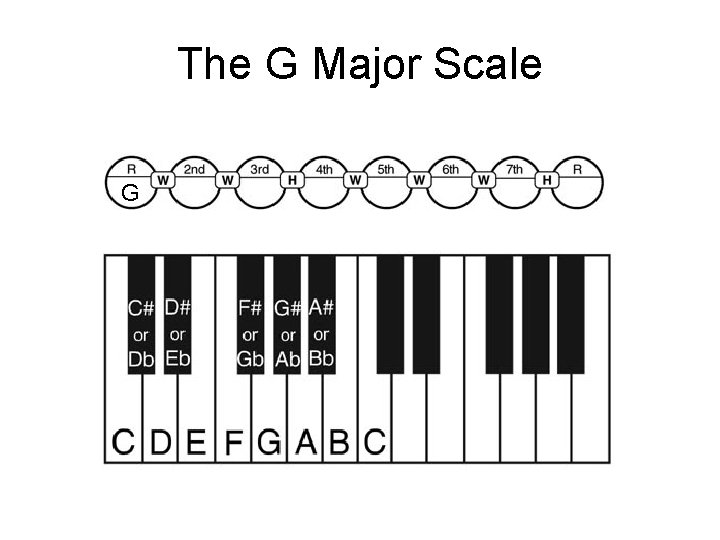The G Major Scale G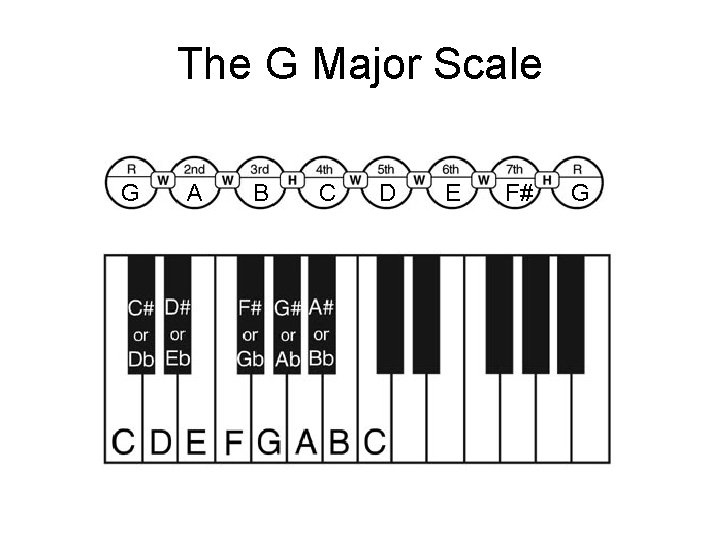The G Major Scale G A B C D E F# G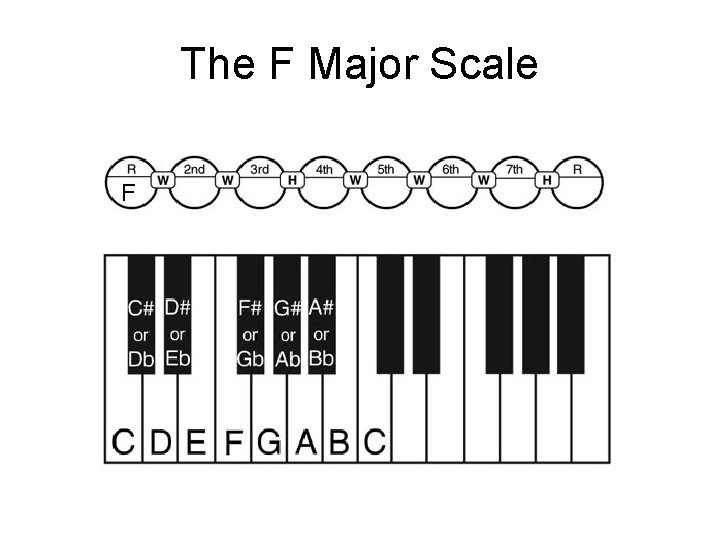The F Major Scale FThe F Major Scale F G A Bb C D E F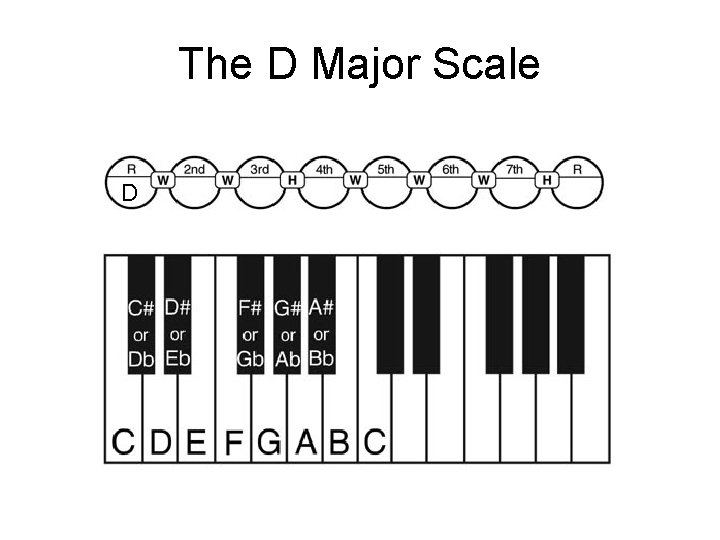The D Major Scale DThe D Major Scale D E F# G A B C# D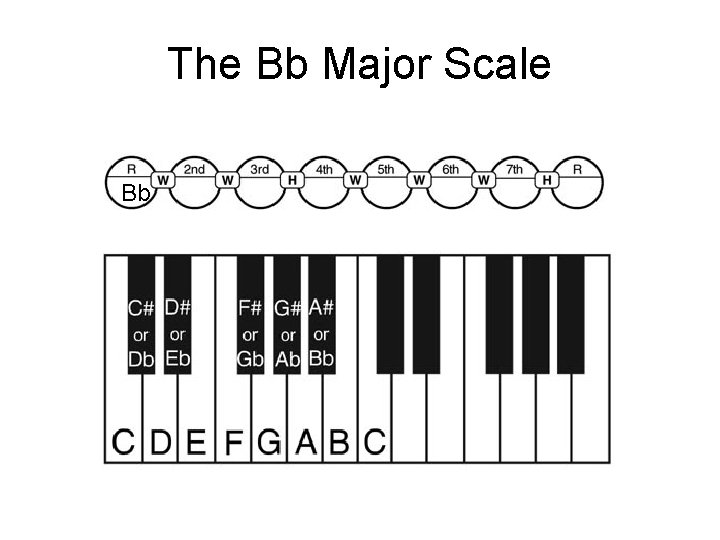The Bb Major Scale Bb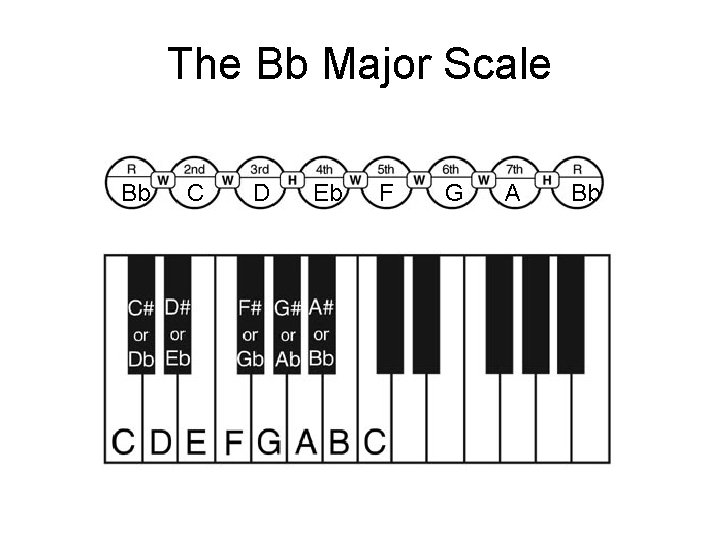The Bb Major Scale Bb C D Eb F G A Bb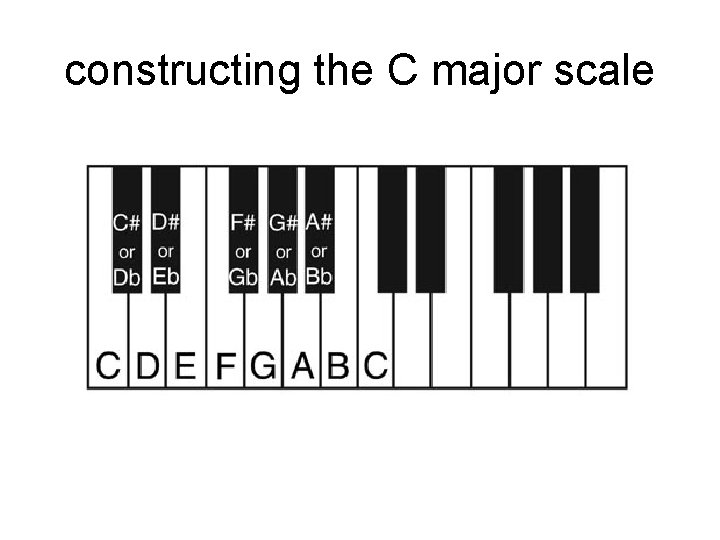constructing the C major scale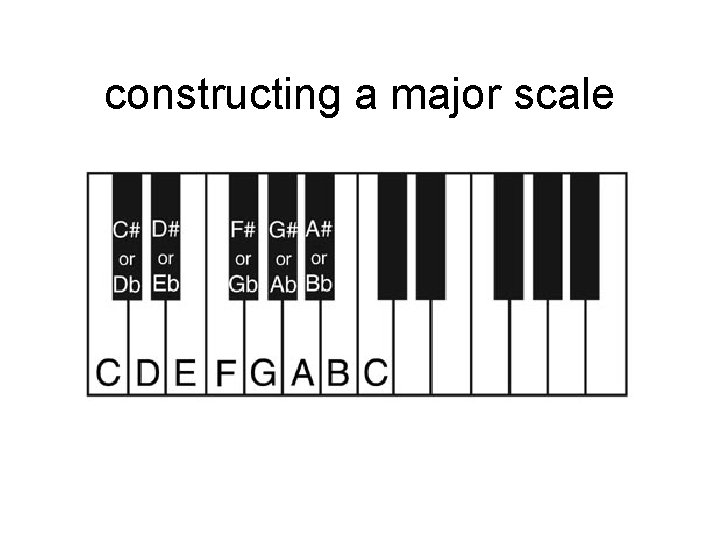constructing a major scale